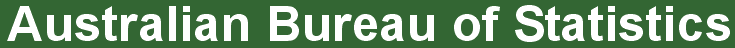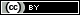ABS Home
 Statistical Language - Estimate and ProjectionEstimate and Projection

What is an estimate?

An estimate is a value that is inferred for a population based on data collected from a sample of units from that population. Estimation is a technique that systematically adjusts the sample data to determine an estimated value for the population.

For example, if our sample data shows that 51% of the sample are female, then the population value will be estimated to be 51% (as estimation is based on the assumption that the sample is representative of the population).

An estimate is not a guess, it is a value based on sampled data which has been adjusted using statistical estimation procedures.

What is a projection?

A projection indicates what the future changes in a population would be if the assumptions about future trends actually occur. These assumptions are often based on patterns of change which have previously occurred.

For example: Data collected about the total number of store locations for a retail chain over three years show an increase from 8 stores in first year, to 12 stores in the second year, to 18 stores in the third year. It could therefore be projected that if the chain continues to expand following the same pattern of increasing by half (50%) each year there will be 27 stores after the fourth year.

A projection is not making a prediction or forecast about what is going to happen, it is indicating what would happen if the assumptions which underpin the projection actually occur.

Comparison of Projections and Forecasts

 Type of Information The Difference Nature of Assumptions Projections indicate what future values for the population would be if the assumed patterns of change were to occur. They are not a prediction that the population will change in this manner. While both involve analysis of data, the key difference between a forecast and a projection is the nature of the assertion in relation to the assumptions occurring. A projection simply indicates a future value for the population if the set of underlying assumptions occur. Forecasts speculate future values for the population with a certain level of confidence, based on current and past values as an expectation (prediction) of what will happen. In a forecast, the assumptions represent expectations of actual future events.

How do estimates and projections differ?

An estimate is a statistic about a whole population for a previous reference period which is based on data from a sample of the population, whereas a projection is a statistic indicating what a value would be if the assumptions about future trends hold true (often drawing upon past movements in a population as a guide for the assumptions).

Further information

ABS:
Animated Population Pyramid
3222.0 - Population Projections, Australia, 2006 to 2101
3228.0.55.001 - Population Estimates: Concepts, Sources and Methods
Health - If Australia were only 100 people...

 Privacy | Disclaimer | Feedback || © Copyright| Sitemap| Online Security